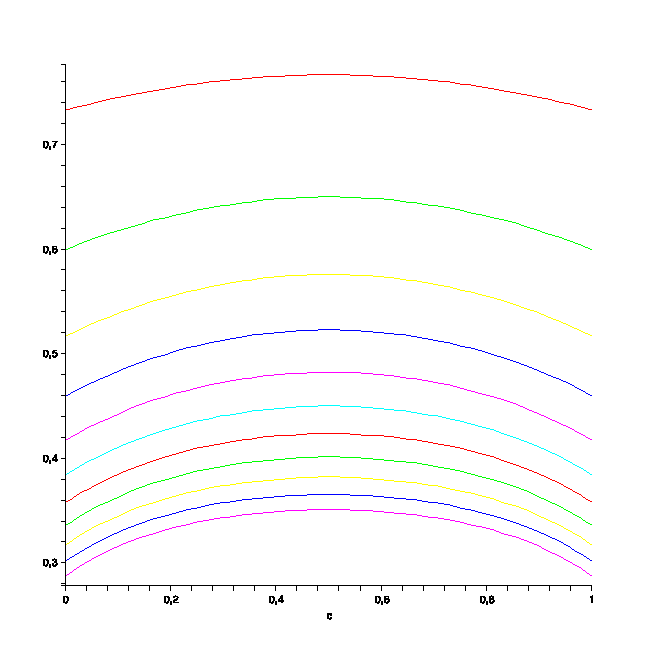# Probability density that minimizes the sample range

Let $\mathcal{F}$ denote the set of all "concave probability distributions" on the unit interval, that is, all functions $f:[0,1]\to \mathbb{R}$ such that $f$ is concave, $f(x)\geq 0$ for all $x\in [0,1]$, and $\int_0^1 f(x) dx=1$. Given a collection of $n$ samples $X_1 , \dots, X_n$, define $R(n) = \mathbf{E}( \max_i X_i - \min_i X_i )$ to be the expected length of the sample range of the samples $X_n$. My question is: what is the density $f^* \in \mathcal{F}$ that minimizes $R(n)$? If that's too complicated, is there a useful lower bound for $R(n)$?

• What about the density $f$ being a triangle-shaped function centered at $1/2$, of width $2\epsilon$ and height $1/\epsilon$? Then the range of a sample is almost surely less than $2\epsilon$. – Eckhard Jan 28 '13 at 16:27

For $n$ i.i.d. random variables $X_1,\dots,X_n$ with (cumulative) distribution function $F(x)= \int _ 0 ^ x f(t)dt\,$ we have $\mathbb{P}(\max_ {1\le i \le n} X _i \le x) = F(x)^n \,$, $\mathbb{P}(\min _ {1\le i \le n} X _ i \le x) = 1 - \big ( 1- F(x)\big) ^n \, .$ Therefore the random variables $\max _ {1\le i \le n} X _ i$ and $\max _ {1\le i \le n} X _i$ have density functions respectively $\big( F(x)^n\big)'$ and $\big(1 - \big (1- F(x)\big )^n\big)'\, .$ Therefore

$$\mathbb{E} \big ( \max _ {1\le i \le n} X _ i - \min _ {1\le i \le n} X _ i\big ) =\mathbb{E} \big (\max_ {1\le i \le n} X _i \big )- \mathbb{E} \big (\min _ {1\le i \le n} X _ i\big ) =$$ $$\int_0^1 x\bigg( F ^ n + \big( 1-F \big)^ n \bigg)'dx = 1 - \int_0^1 \bigg( F ^ n + \big( 1-F \big)^ n \bigg)dx =\int _ 0 ^ 1 p\big(F(x)\big)dx \, ,$$ after integration by parts; here $p(t)$ is the strictly concave polynomial function $$p(t):= 1- t^n -(1-t)^n\, .$$

$$*$$

We have therefore to minimize the strictly concave functional $$J(f):=\int _ 0 ^ 1 p\Big( \int _ 0 ^ x f dt\Big) dx \, ,$$ on the convex compact subset (see 1 below) $K\subset L^1([0,1])$ of all positive, concave, norm-one functions $f$. A continuous strictly concave functional defined on a compact convex set $K$ attains its infimum on the extreme points of $K$. Here (see 2 below), the extreme points of $K$ are exactly the family of piecewise linear functions, for $\{f_c \}_ {0\le c\le 1}$,

$$f_c(x):= \frac{2x}{c}\mathrm{\quad if\quad } 0\le x\le c\, ,$$ $$f_c(x):= \frac{2(1-x)}{1-c} \mathrm{\quad if\quad } c\le x\le 1\, .$$ The minimization problem is therefore reduced to the computation of the one-variable maximum: $$\min _ {f\in K} J(f )=\min _ {0\le c \le 1} J(f_c) =1-\max _ {0\le c \le 1} g(c)\, ,$$

where $g(c)= 1-J(f_c)$ is $$g(c) =\int_0^1 \bigg( c^{n+1}x^{2n} + (1-c)^{n+1}x^{2n} + c( 1- cx^2 )^n +(1-c)\big( 1- (1-c)x^2 \big)^n \bigg) dx \, ;$$ so $g$ is a polynomial in $c$ symmetrical w.r.to $c=1/2$. It should be true that $g$ is concave in $c$, so that one eventually finds $$\min _ {f\in K} J(f )=1 -\frac{1}{2^n(2n+1)} - \int_0^1 \bigg( 1- \frac{x^2}{2} \bigg)^n dx \, .$$

For $n\to +\infty$ this is (see 3 below) $$1- \sqrt{\frac{\pi}{2n}} + o\Big(\frac{1}{n}\Big)\, .$$Plots of the polynomials $g(c)$, for $n=2,\dots,12$, from top to bottom.

$$*$$

More details.

1. (Compactness of $K$). Note that by definition of $K$, each $f\in K$ satisfies $$0 \le f(x) \le 2\\ ,$$ for all $x\in [0,1]$ and, for any $\epsilon > 0$, $$\mathrm{Lip}\big(f,\\ [\epsilon, 1-\epsilon]\big) \le \frac{2}{\epsilon} \, ,$$ which is sufficient to conclude that $K$ is compact in $L^1$ by the Ascoli-Arzelà and the dominated convergence theorems.

2. (Extreme points of $K$). Note that for any $f\in K$ and $c\in [0,1]$, we have $f=f_c$ if and only if $f(c)\ge 2$. This implies that all these $f_c$'s are extreme points of $K$ (if $f_c$ is an arithmetic mean of two elements of $K$, at least one of them has value at $c$ not less than $2$, hence it is $f_c$ itself). Conversely, if $f\in K$ has $\|f\|_\infty < 2$, then one can find a non-zero function $h$ with $\int_0^1 hdx =0$ and such that $f\pm h$ are concave and non-negative, hence in $K$, so that $f =\frac{1}{2}\big((f+h)+(f-h)\big)$ is not extremal.

3. (Asymptotics). Incidentally, a quick asymptotics for $\int_0^1 \big( 1- \frac{x^2}{2} \big) ^ n dx$ as $n\to\infty$ comes from the dominated convergence theorem, recalling that $0\le (1+t/n)^n\le e^t$ as soon as $1+t/n\ge0$. Changing variable, $x:=\xi/\sqrt n$,

$$\int_0^1 \bigg( 1- \frac{x^2}{2} \bigg) ^ n dx = \sqrt{\frac{1}{n}} \int _ 0 ^{+\infty} \bigg( 1- \frac {\xi^2}{2n} \bigg) ^ n \chi _ {[0,\sqrt n]}(\xi) d\xi$$

$$= \sqrt{\frac{1}{n}} \int _ 0 ^{+\infty} e^ { -\xi^2/2 } d\xi \, \big(1+ o(1)\big) = \sqrt{\frac{\pi}{2n}}+ o(\frac{1}{n})\, .$$

• thank you, plugging this into maple suggests that $R \sim 1-n^{-2}$ and that the optimal $c$ is $1/2$! – Charlie Jan 28 '13 at 20:46
• I agree, I added some more details. – Pietro Majer Jan 29 '13 at 9:30
• (by the way, I found R∼ 1−√(π/2n) , e.g. for n=10, R(10)=0.61.. and 1−√(π/20)=0.60..) – Pietro Majer Jan 30 '13 at 3:26